# Independent Samples T-Test - Pooled Variance

Where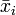$\bar{x}_i$ is the mean of variable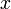$x$ for the$i$th of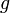$g$ groups,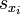$s_{x_i}$ is its Standard Deviation and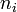$n_i$ its sample size, the test statistic is for the difference between groups$i$ and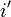$i'$ is given by: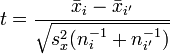$t=\frac{\bar{x}_i-\bar{x}_{i'}}{\sqrt{s^2_x(n^{-1}_i + n^{-1}_{i'})}}$,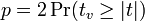$p = 2\Pr(t_v \ge |t|)$,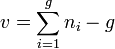$v = \sum^g_{i=1} n_i - g$, and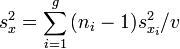$s^2_{x} = \sum^g_{i=1}{ ( n_i - 1 ) s^2_{x_i}} / v$.